# Plotting lines

Plotting lines is handled by `pygmt.Figure.plot`.

```import pygmt
```

## Plot lines

Create a Cartesian figure using `projection` parameter and set the axis scales using `region` (in this case, each axis is 0-10). Pass a list of `x` and `y` values to be plotted as a line.

```fig = pygmt.Figure()
fig.plot(
region=[0, 10, 0, 10],
projection="X15c/10c",
frame="a",
x=[1, 8],
y=[5, 9],
pen="1p,black",
)
fig.show()
```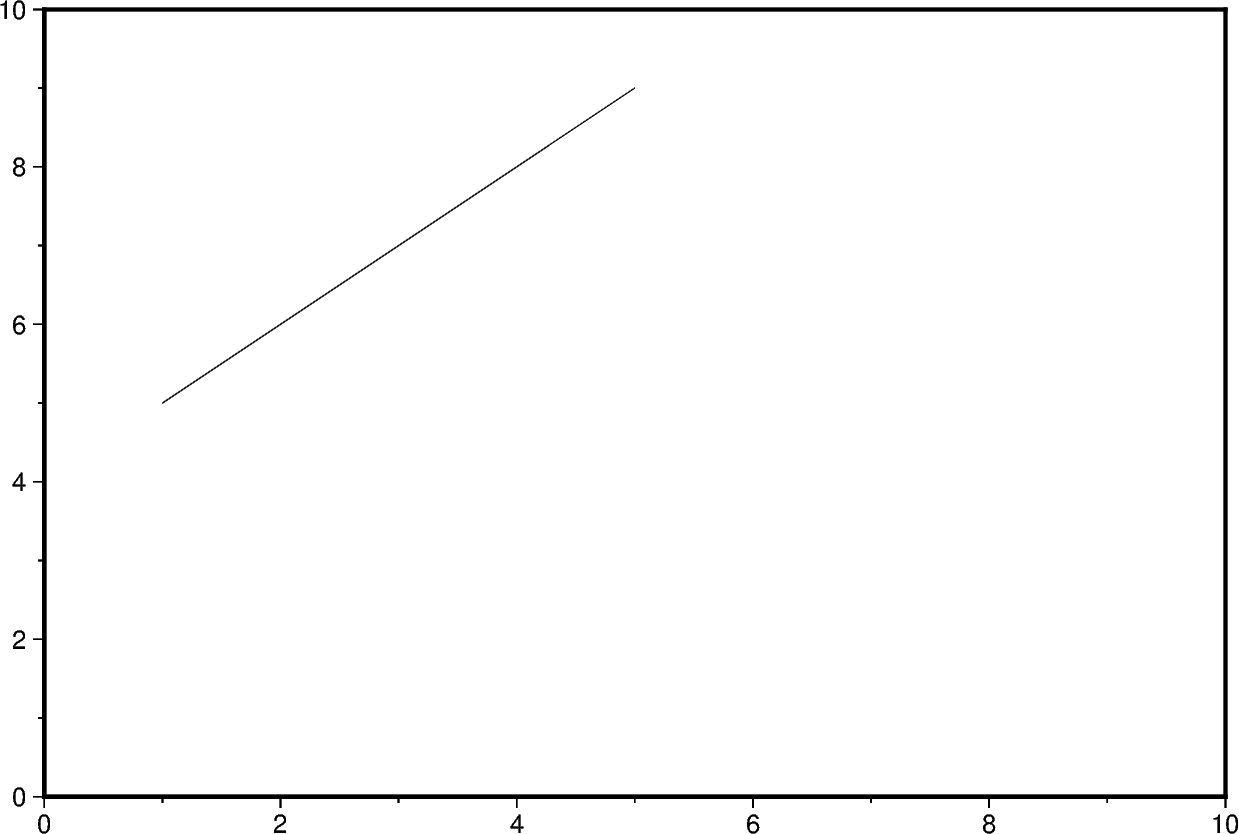Out:

```<IPython.core.display.Image object>
```

Additional line segments can be added by including additional values for `x` and `y`.

```fig = pygmt.Figure()
fig.plot(
region=[0, 10, 0, 10],
projection="X15c/10c",
frame="a",
x=[1, 6, 9],
y=[5, 7, 4],
pen="1p,black",
)
fig.show()
```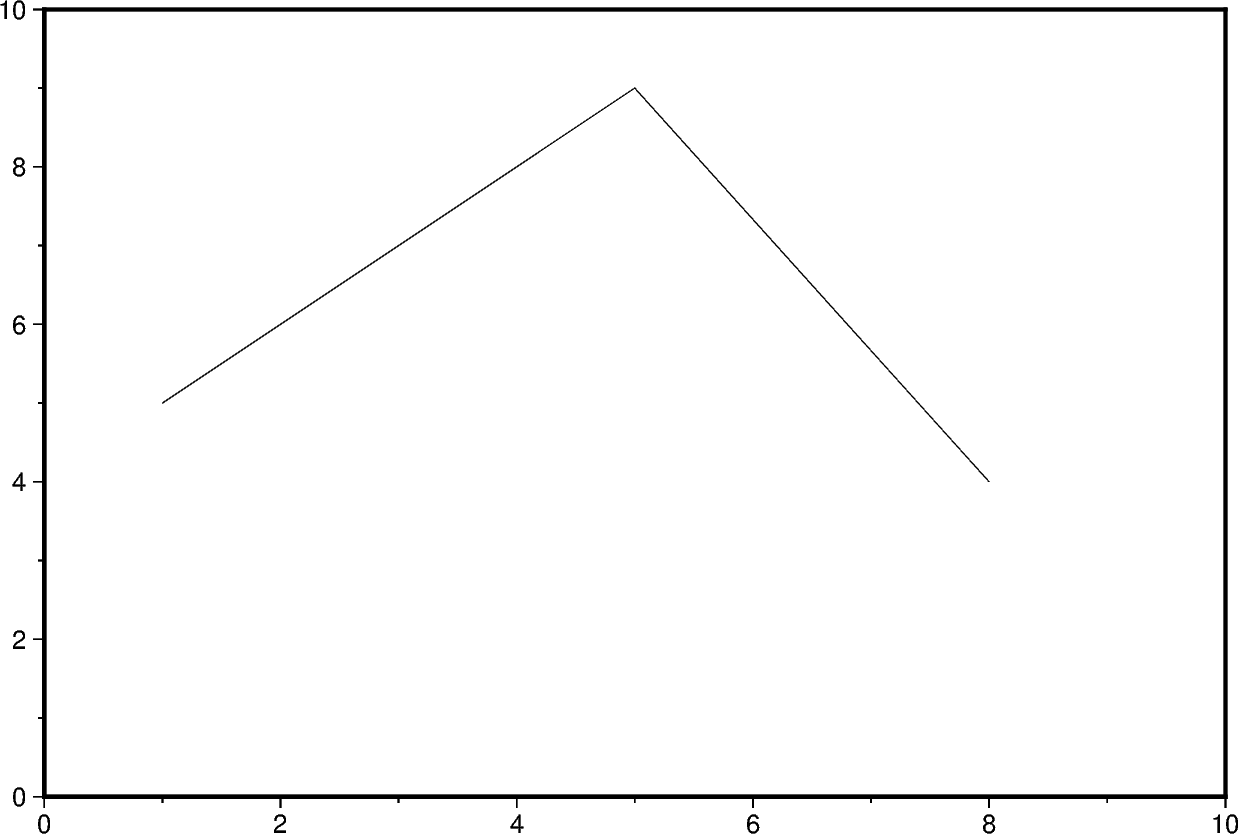Out:

```<IPython.core.display.Image object>
```

To plot multiple lines, `pygmt.Figure.plot` needs to be used for each additional line. Arguments such as `region`, `projection`, and `frame` do not need to be repeated in subsequent uses.

```fig = pygmt.Figure()
fig.plot(
region=[0, 10, 0, 10],
projection="X15c/10c",
frame="a",
x=[1, 6, 9],
y=[5, 7, 4],
pen="2p,blue",
)
fig.plot(x=[2, 4, 10], y=[3, 8, 9], pen="2p,red")
fig.show()
```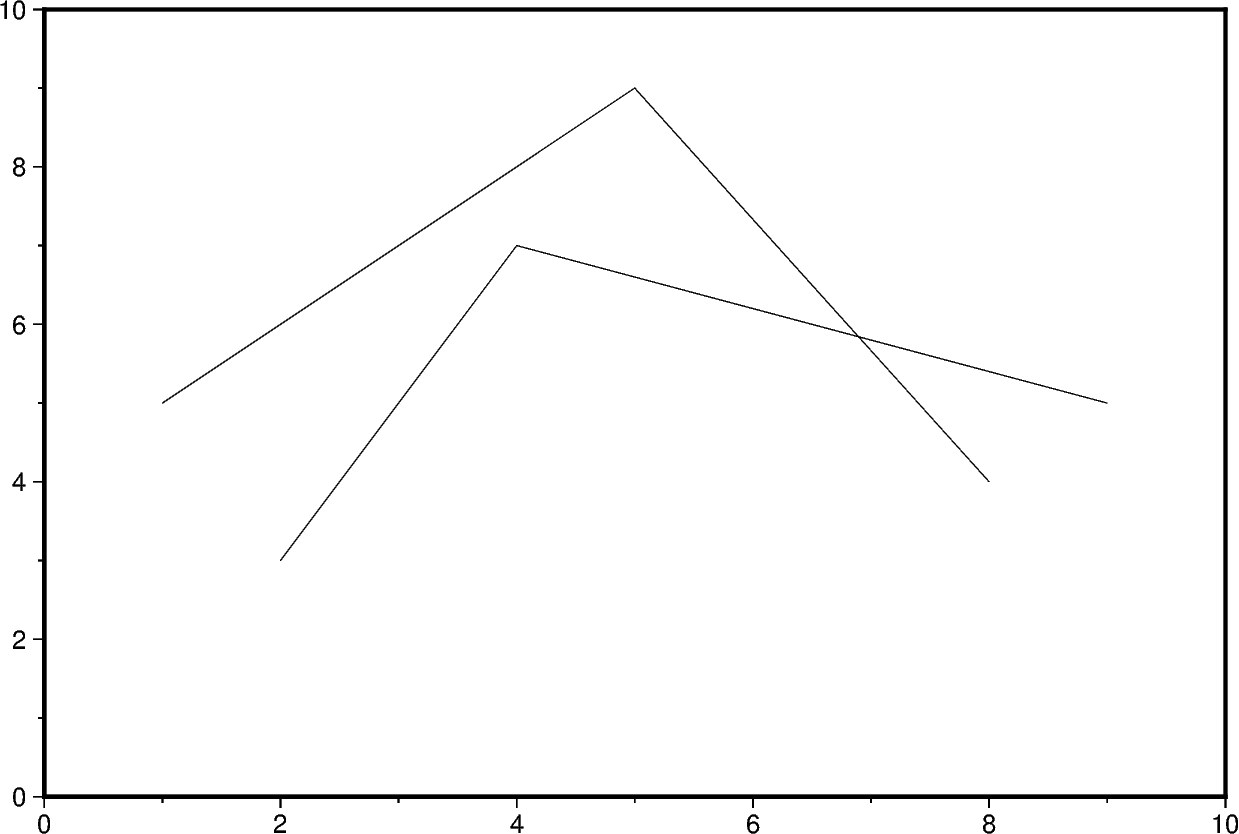Out:

```<IPython.core.display.Image object>
```

## Change line attributes

The line attributes can be set by the `pen` parameter. `pen` takes a string argument with the optional values width,color,style.

In the example below, the pen width is set to `5p`, and with `black` as the default color and `solid` as the default style.

```fig = pygmt.Figure()
fig.plot(
region=[0, 10, 0, 10],
projection="X15c/10c",
frame="a",
x=[1, 8],
y=[3, 9],
pen="5p",
)
fig.show()
```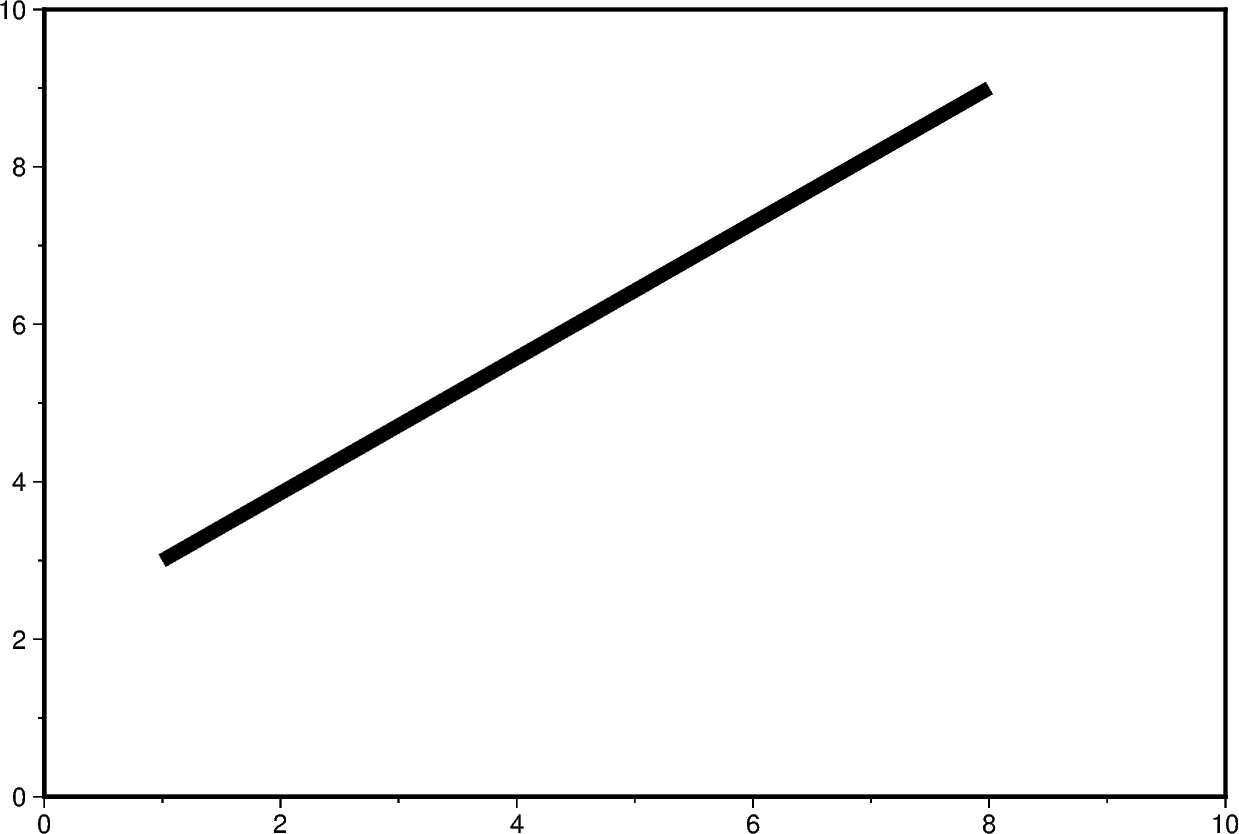Out:

```<IPython.core.display.Image object>
```

The line color can be set and is added after the line width to the `pen` parameter. In the example below, the line color is set to `red`.

```fig = pygmt.Figure()
fig.plot(
region=[0, 10, 0, 10],
projection="X15c/10c",
frame="a",
x=[1, 8],
y=[3, 9],
pen="5p,red",
)
fig.show()
```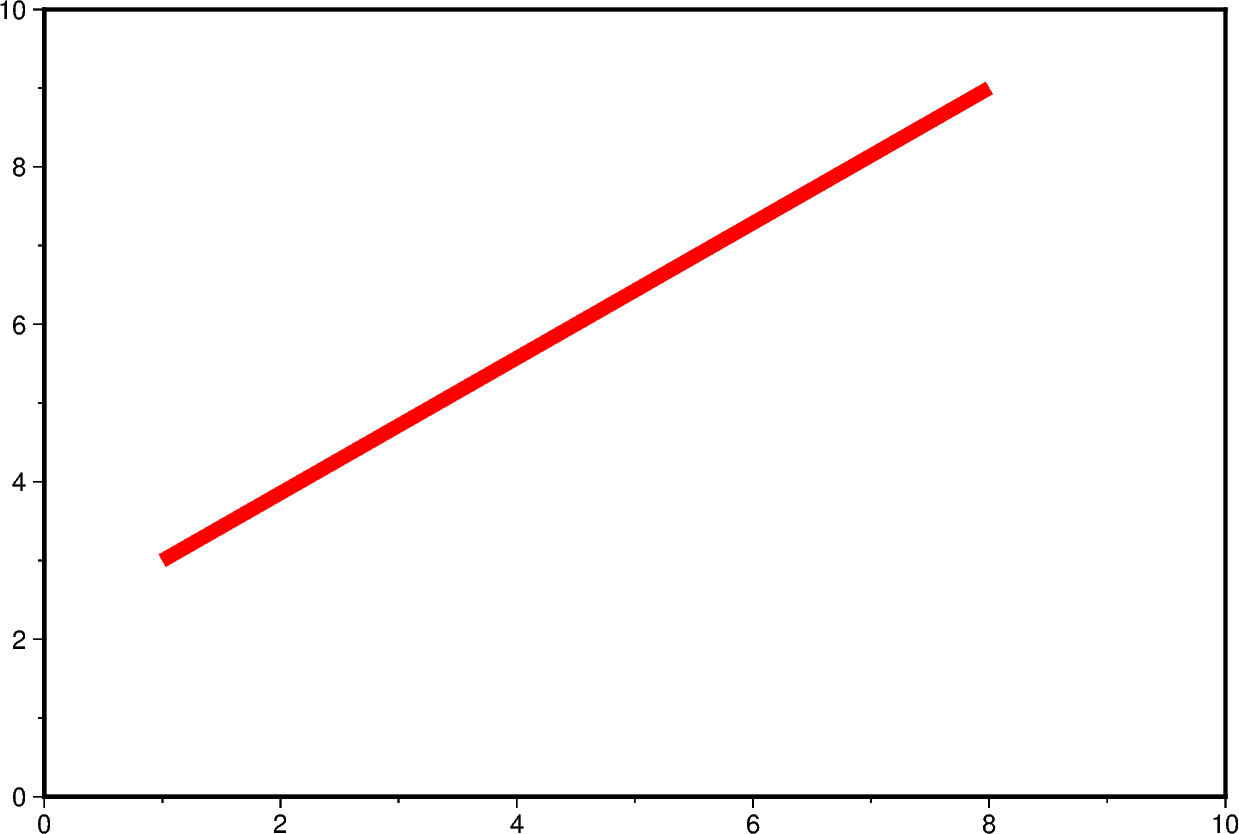Out:

```<IPython.core.display.Image object>
```

The line style can be set and is added after the line width or color to the `pen` parameter. In the example below, the line style is set to `..-` (dot dot dash), and the default color `black` is used.

```fig = pygmt.Figure()
fig.plot(
region=[0, 10, 0, 10],
projection="X15c/10c",
frame="a",
x=[1, 8],
y=[3, 9],
pen="5p,..-",
)
fig.show()
```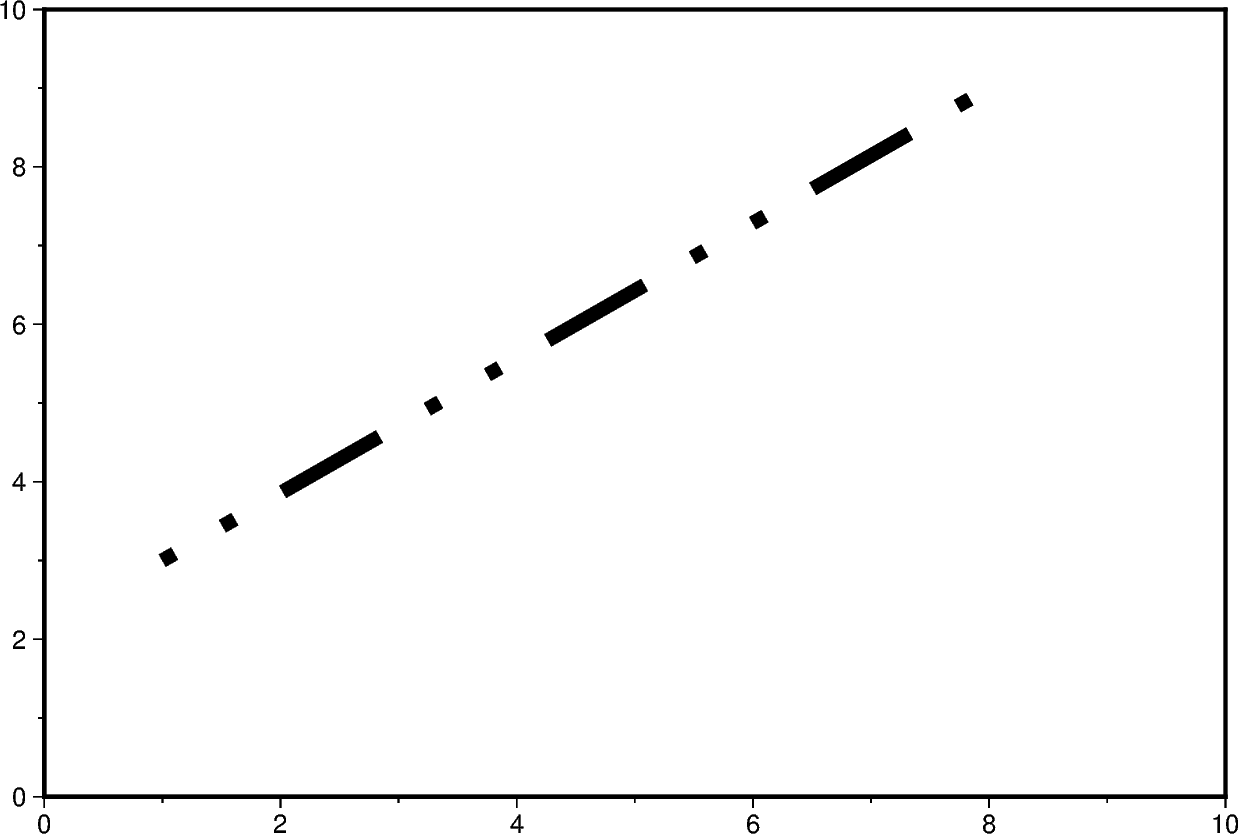Out:

```<IPython.core.display.Image object>
```

The line width, color, and style can all be set in the same `pen` parameter. In the example below, the line width is set to `7p`, the color is set to `green`, and the line style is `-.-` (dash dot dash).

For a gallery showing other `pen` settings, see Line styles.

```fig = pygmt.Figure()
fig.plot(
region=[0, 10, 0, 10],
projection="X15c/10c",
frame="a",
x=[1, 8],
y=[3, 9],
pen="7p,green,-.-",
)
fig.show()
```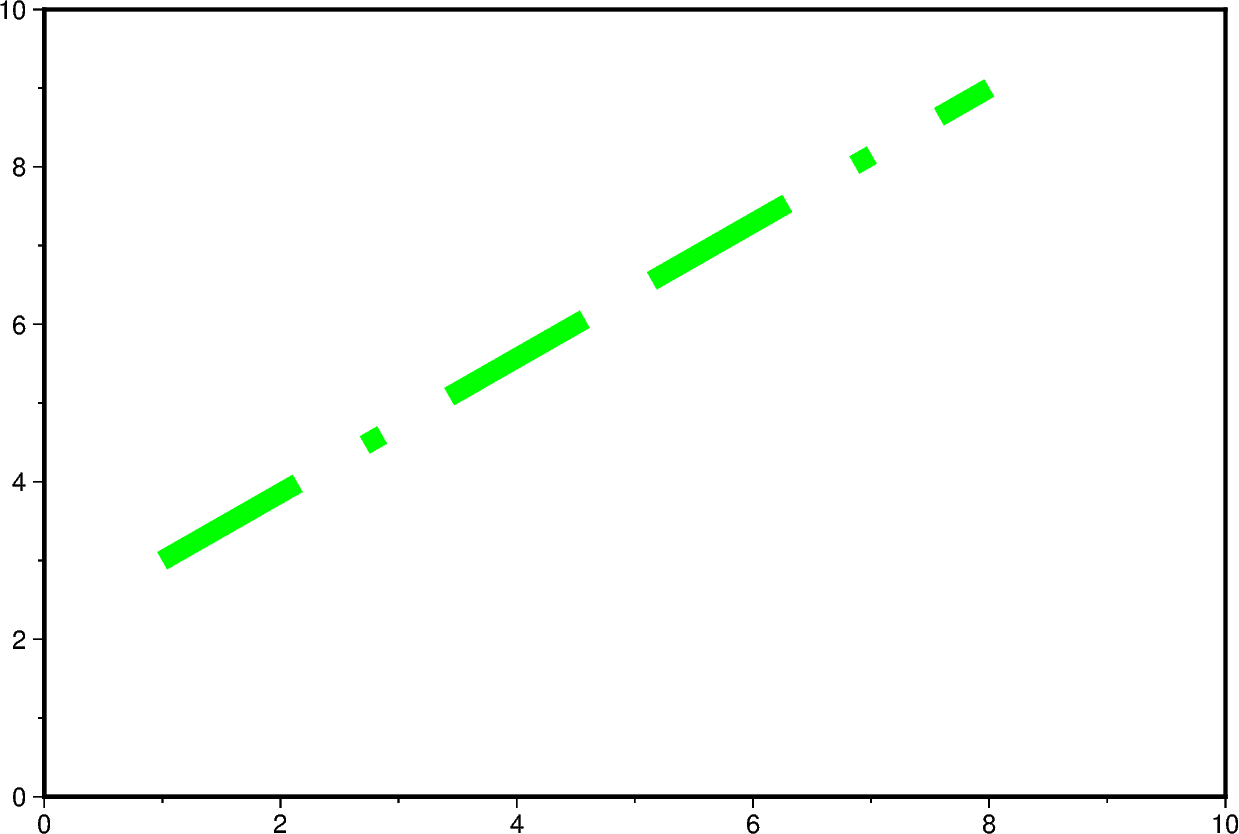Out:

```<IPython.core.display.Image object>
```

Total running time of the script: ( 0 minutes 5.608 seconds)

Gallery generated by Sphinx-Gallery